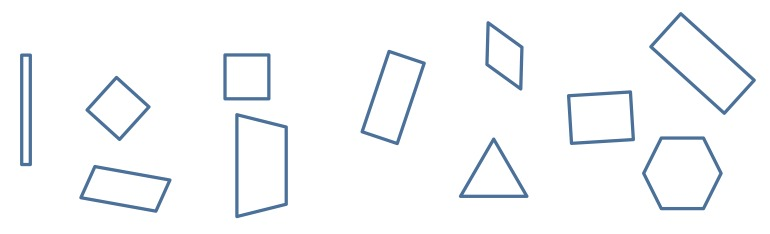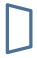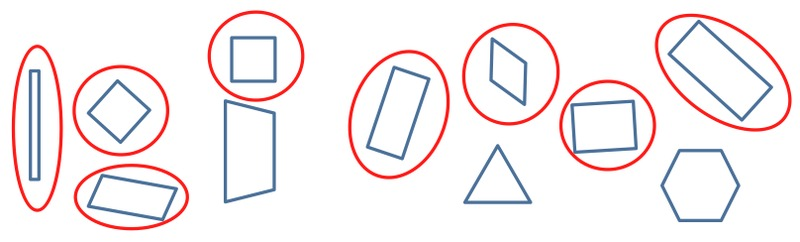# Defining Attributes of Rectangles and Parallelograms

Alignments to Content Standards: 4.G.A.2

1. Look at each figure. Read each of the descriptions. Place an X in the box if it appears to describe the figure pictured.

4 vertices Four sides Opposite sides parallel Perpendicular sides A.B.C.D.2. What is true of all the figures?

3. All of the figures above are rectangles. Ring the rectangles below. Using what you know that is true of all rectangles to help you.Choose one figure you did not ring. Explain why it is not a rectangle.

Look at each figure. Read each of the attributes. Place an X in the box if it appears to be an attribute of the figure pictured.What is true of all the figures?

All of the figures above are parallelograms. Ring all the parallelograms below. Using what you know that is true of all parallelograms to help you.Choose one figure you did not ring. Explain why it is not a parallelogram.

## IM Commentary

At early stages of understanding of geometric shapes, students describe shapes, but do not yet characterize shapes by their properties. One example of a misconception students have at this stage is that a rectangle has two short sides and two long sides.

The purpose of this task is for students to identify the defining attributes of rectangles and parallelograms. This task should be completed after students have many experiences sorting shapes by attributes. Understanding which characteristics are defining attributes will allow students to use work toward 5th grade 5.G.3 (Understand that attributes belonging to a category of two-dimensional figures also belong to all subcategories of that category) and 5.G.4 (Classify two-dimensional figures in hierarchy based on properties.)

This task could be altered to identify defining attributes of triangles and other quadrilaterals.

## SolutionWhat is true of all the figures?

All of the figures have 4 sides and 4 vertices with opposite sides parallel and congruent. They have four right angles, so they also have perpendicular line segments.

All of the figures above are rectangles. Put a ring around all the rectangles below. Using what you know that is true of all rectangles to help you.Choose one figure you did not circle. Explain why it is not a rectangle.

Example: This figurehas 4 sides and 4 vertices. Only one set of opposite sides are parallel and it does not have right angles, so it cannot be a rectangle.What is true of all the figures?

All the figures have 4 sides and 4 vertices with opposite sides parallel and congruent.

All of the figures above are parallelograms. Put a ring around all the parallelograms below. Using what you know that is true of all parallelograms to help you.Choose one figure you did not ring. Explain why it is not a parallelogram.

Example: The regular hexagon has opposite sides parallel and congruent, but it is not 4-sided.